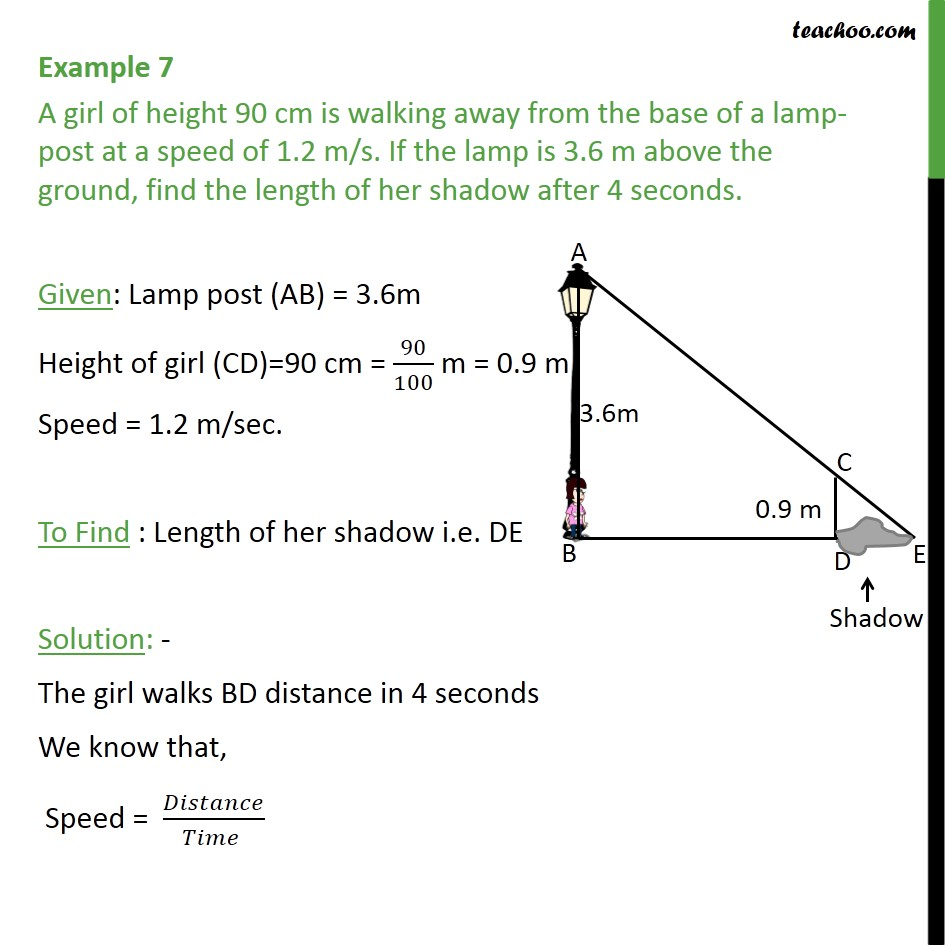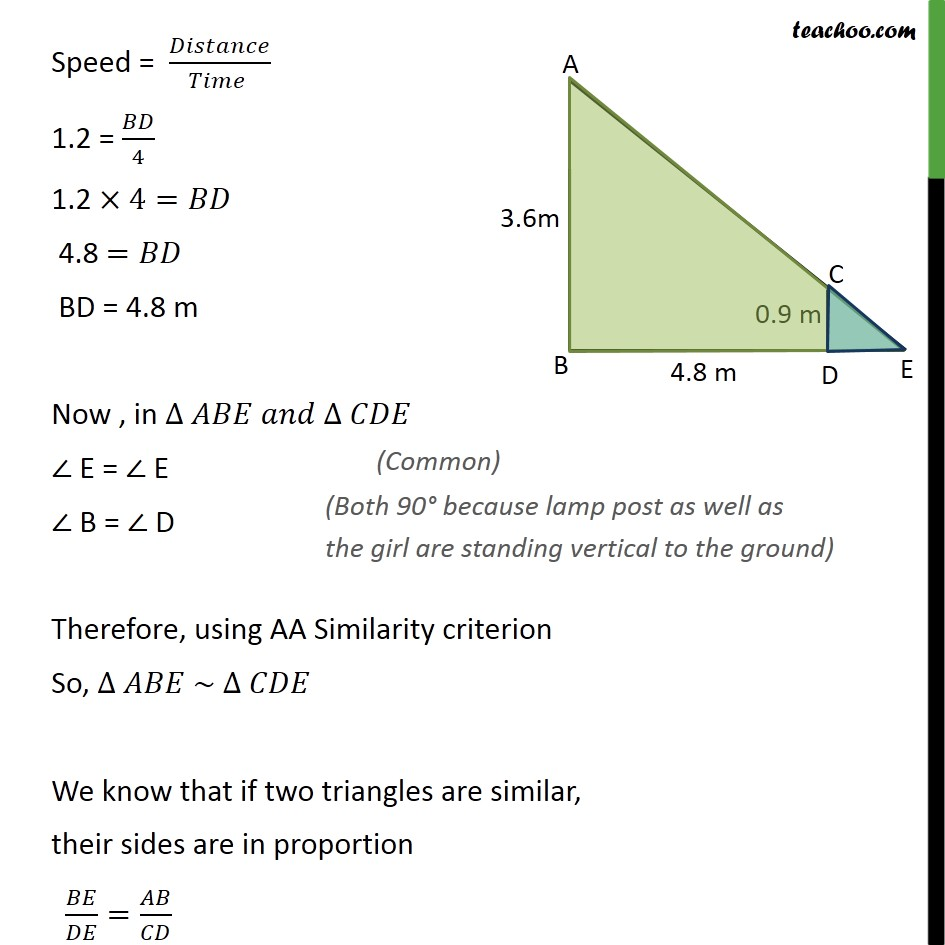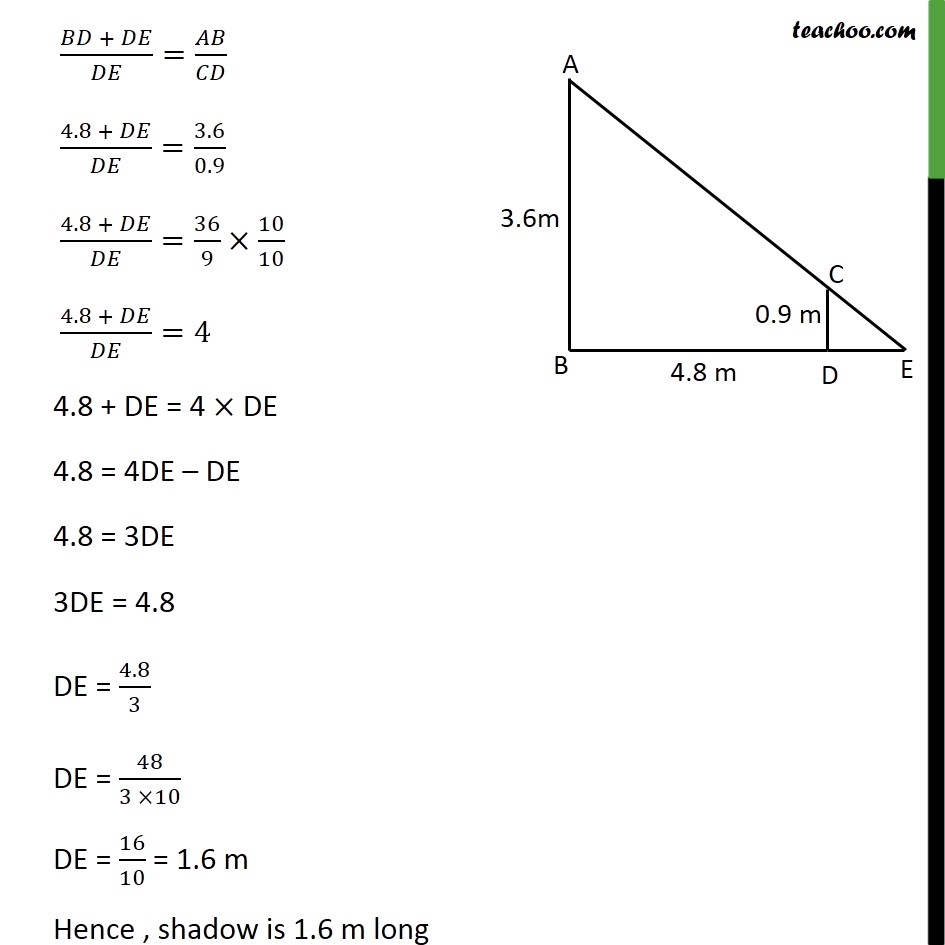Subscribe to our Youtube Channel - https://you.tube/teachoo

1. Chapter 6 Class 10 Triangles
2. Concept wise
3. AA Similarity

Transcript

Example 7 A girl of height 90 cm is walking away from the base of a lamp-post at a speed of 1.2 m/s. If the lamp is 3.6 m above the ground, find the length of her shadow after 4 seconds. Given: Lamp post (AB) = 3.6m Height of girl (CD)=90 cm = 90/100 m = 0.9 m Speed = 1.2 m/sec. To Find : Length of her shadow i.e. DE Solution: - The girl walks BD distance in 4 seconds We know that, Speed = /( ) Speed = /( ) 1.2 = /4 1.2 4= 4.8 = BD = 4.8 m Now , in E = E B = D Therefore, using AA Similarity criterion So, ~ We know that if two triangles are similar, their sides are in proportion / = / ( + )/ = / (4.8 + )/ =3.6/0.9 (4.8 + )/ =36/9 10/10 (4.8 + )/ =4 4.8 + DE = 4 DE 4.8 = 4DE DE 4.8 = 3DE 3DE = 4.8 DE = 4.8/3 DE = 48/(3 10) DE = 16/10 = 1.6 m Hence , shadow is 1.6 m long

AA Similarity